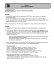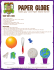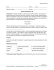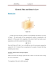# Document 6537223

## Transcription

Document 6537223
```Physics 1B
Quiz 1 Sample Questions
(1) A single point charge is placed at the origin. Which of the following statements is true?
Assume the locations in the answers all lie on the x-axis (i.e. y=0.0 m).
(a) The magnitude of the electric field at x=2.0m is the same as the magnitude of the
electric field at x=-2.0m.
(b) The magnitude of the electric field at x=2.0m is twice the magnitude of the
electric field at x=4.0m.
(c) The magnitude of the electric field at x=2.0m is four times the magnitude of the
electric field at x=4.0m.
(d) Both (a) and (b) are true.
(e) Both (a) and (c) are true.
Questions 2 and 3 refer to the following situation: a charge of +5.0 C is placed at x = -2.0 m.
A second charge of +5.0 C is placed at the origin. The location labeled P has coordinates
x = -1.0 m, y = -1.0 m.
(2) The magnitude of the net electric field at P is
(a) 3.2 x 1010 N/C
(b) 4.5 x 1010 N/C
(c) 5.6 x 1010 N/C
(d) 2.2 x 1010 N/C
(e) 0
(3) The direction of the net electric field at P
(a)
(b)
(c)
(d)
(e)
in the direction of the positive y-axis.
in the direction of the negative y-axis.
in the direction 45° below the positive x-axis.
in the direction 45° below the negative x-axis.
there will be no direction because the net electric field is zero at point P.
(4) A positive point charge is placed inside a non-spherical rubber balloon. The balloon is
originally neutral and the point charge never touches the balloon. The point charge is
located at the center of the balloon. Which of the following is true?
(a) The electric flux through the balloon would increase if the balloon was blown up
more.
(b) The electric field has the same strength everywhere on the surface of the balloon.
(c) The electric flux through the balloon changes if the point charge moves to a new
position inside the balloon.
(d) The net charge of the balloon itself is zero.
(e) All of the above are true.
(5) Two charges are fixed at (-4, 0) and (+4, 0) as shown in the figure: q1 = +2 µC and q2 = 2 µC. What is the magnitude of the electric field at point P [with coordinate (0, 2)] due to
charges q1 and q2?
(a)
(b)
(c)
(d)
(e)
1798 N/C
1608 N/C
899 N/C
804 N/C
0 N/C
(6) A neutral conducting sphere is placed midway between two fixed unequal point charges
q1 = +4 µC and q2 = -2 µC. The sphere is free to move. In which direction do you expect
it to move?
(a) to the left
(b) to the right
(c) The sphere will not move.
(7) The picture below shows an electron about to enter a region with an electric field. The
velocity of the electron is denoted with the dashed arrow.
What will be the direction of the electrostatic force the electron feels as it first enters the
region with the E-field? (a)
(a)
(b)
(c)
(d)
(e)
To the left
To the right
Up and to the right
Down and to the left
There will be no force
(8) Two small spheres with equal unknown mass are suspended by light-weight strings of
length 12 cm. When a charge of
+3 nC is placed on each sphere,
each string makes an angle of
20° with the vertical in
equilibrium.
What is the mass of each ball?
(a)
(b)
(c)
(d)
(e)
1.21 × 10-6 kg
3.37 × 10-6 kg
1.07 × 10-5 kg
1.35 × 10-5 kg
2.23 × 10-5 kg
(9) Three charges (-q, -q, +3q) are located in the xy plane as shown in the figure. A charge
2q is brought in as below. What is the x-component of the force felt by the charge +2q
in its new position?
(a)
(b)
(c)
(d)
(e)
(10)
Fx = -10.8 N
Fx = -7.6 N
Fx = 0 N
Fx = +7.6 N
Fx = +10.8 N
Given are 4 charges on a square of side a = 25 cm (see figure above). q = 1 µC.
What is the x component of electric field at the center of the square (Ex)?
(a)
(b)
(c)
(d)
(e)
\
Ex = -611,000 N/C
Ex = -204,000 N/C
Ex = 0 N/C
Ex = 204,000 N/C
Ex = 611,000 N/C
(11)
The conducting sphere of an electroscope is loaded with charge q so that the
conducting leaves stand apart as shown in Figure I. As a rod with charge QA is brought
near (but does not touch) the conducting sphere in figure II, the leaves move towards one
another. This rod is removed and a different rod holding charge QB is brought near the
conducting sphere in Figure III. The leaves are seen to move farther apart than in Figure
I.
Which one of the following statements must be true?
(a)
(b)
(c)
(d)
(e)
The magnitude of QA is less than the magnitude of q.
QA and q have the same sign.
QB and q have the same sign.
QA is positive and QB is negative.
QA is negative and QB is positive.
(12)
The total outward electric flux through the closed cylindrical surface (length 1.2
m, diameter 0.2 m) is equal to -5.0 Nm2/C. Determine the net charge Q within the
cylinder.
(a) Q = - 53 pC
(b) Q = - 44 pC
(c) Q = + 53 pC
(13)
The electric field in a region of space is described by the electric field line pattern
shown. The two small "x" denote two different points in the field, labeled A and B.
Where is the electric field stronger?
(a) Point A
(b) Point B
(c) They are equal
```

### Assignment (02) Introduction to Electric Charges and Electric Field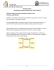### Colorfest Poster.indd - Pagosa Springs Chamber of Commerce### Schedule of Events - 2016 Teton Valley Balloon Rally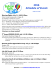### these are some in our guestbook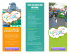### Balloon - Ultra Furniture Warehouse### Schedule of Events - 2016 Teton Valley Balloon Rally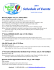### File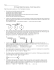### Conwin Declaration of Conformity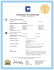### Sample Test MT1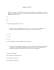### Electrostatic Force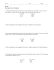### The Fan Club (SR Levels)